Engineering Mechanics - KOP: Work and Energy

Why Engineering Mechanics KOP: Work and Energy?

In this section you can learn and practice Engineering Mechanics Questions based on "KOP: Work and Energy" and improve your skills in order to face the interview, competitive examination and various entrance test (CAT, GATE, GRE, MAT, Bank Exam, Railway Exam etc.) with full confidence.

Where can I get Engineering Mechanics KOP: Work and Energy questions and answers with explanation?

IndiaBIX provides you lots of fully solved Engineering Mechanics (KOP: Work and Energy) questions and answers with Explanation. Solved examples with detailed answer description, explanation are given and it would be easy to understand. All students, freshers can download Engineering Mechanics KOP: Work and Energy quiz questions with answers as PDF files and eBooks.

Where can I get Engineering Mechanics KOP: Work and Energy Interview Questions and Answers (objective type, multiple choice)?

Here you can find objective type Engineering Mechanics KOP: Work and Energy questions and answers for interview and entrance examination. Multiple choice and true or false type questions are also provided.

How to solve Engineering Mechanics KOP: Work and Energy problems?

You can easily solve all kind of Engineering Mechanics questions based on KOP: Work and Energy by practicing the objective type exercises given below, also get shortcut methods to solve Engineering Mechanics KOP: Work and Energy problems.

Exercise :: KOP: Work and Energy - General Questions

• KOP: Work and Energy - General Questions
1.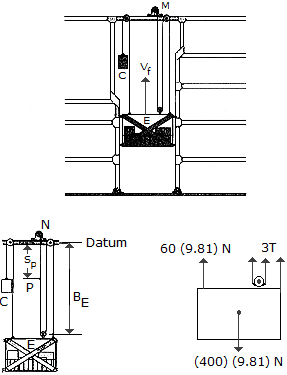The elevator E and its freight have a total mass of 400 kg. Hoisting is provided by the motor M and the 60-kg block C. If the motor has an efficiency of e = 0.6, determine the power that must be supplied to the motor when the elevator is hoisted upward at a constant speed of vE = m/s.

 A. P = 22.2 kW B. P = 13.34 kW C. P = 26.2 kW D. P = 30.1 kW

Explanation:

No answer description available for this question. Let us discuss.

2.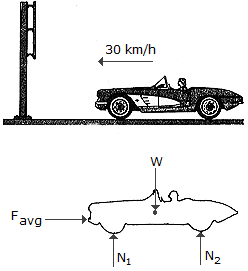A car having a mass of 2 Mg strikes a smooth, rigid sign post with an initial speed of 30 km/h. To stop the car, the front end horizontally deforms 0.2 m. If the car is free to roll during the collision, determine the average horizontal collision force causing the deformation.

 A. Favg = 4500 kN B. Favg = 9000 kN C. Favg = 347 kN D. Favg = 694 kN

Explanation:

No answer description available for this question. Let us discuss.

3.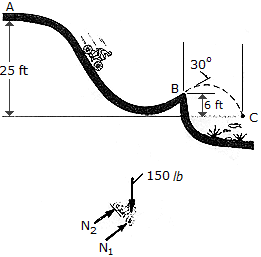When at A the bicyclist has a speed of vA = ft/s. If he coasts without pedaling from the top of the hill at A to the shore of B and then leaps off the shore, determine his speed at B and the distance x where he strikes the water at C. The rider and his bicycle have a total weight of 150 lb. Neglect the size of the bicycle and wind resistance.

 A. vB = 35.0 ft/s, x = 41.2 ft B. vB = 35.0 ft/s, x = 36.1 ft C. vB = 40.1 ft/s, x = 46.5 ft D. vB = 40.1 ft/s, x = 52.0 ft

Explanation:

No answer description available for this question. Let us discuss.

4.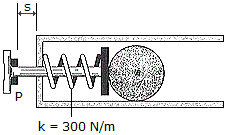The firing mechanism of a pinball machine consists of a plunger P having a mass of 0.25 kg and a spring of stiffness k = 300 N/m. When s = 0, the spring is compressed 50 mm. If the arm is pulled back such that s = 100 mm and released, determine the speed of the 0.3 kg pinball B just before the plunger strikes the stop, i.e., s = 0. Assume all sufaces of contact to be smooth. The ball moves in the horizontal plane. Note that the ball slides without rolling.

 A. v = 4.47 m/s B. v = 3.30 m/s C. v = 2.34 m/s D. v = 3.16 m/s

Explanation:

No answer description available for this question. Let us discuss.

5.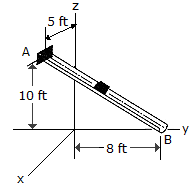The block has a weight of 1.5 lb and slides along the smooth chute AB. It is released from rest at A, which has coordinates of A(5 ft, 0, 10 ft). Determine the speed at which it slides off at B, which has coordinates of B(0, 8 ft, 0).

 A. vB = 28.7 ft/s B. vB = 25.4 ft/s C. vB = 26.8 ft/s D. vB = 29.8 ft/s